meshc

Contour plot under mesh surface plot

Description

example

meshc(X,Y,Z) creates a mesh plot with a contour plot underneath. A mesh plot is a three-dimensional surface that has solid edge colors and no face colors. The function plots the values in matrix Z as heights above a grid in the x-y plane defined by X and Y. The edge colors vary according to the heights specified by Z.

meshc(Z) creates a mesh and contour plot and uses the column and row indices of the elements in Z as the x- and y-coordinates.

example

meshc(___,C) specifies the color of the edges.

meshc(___,Name,Value) specifies additional options for the meshc plot using one or more name-value pair arguments. Specify the options after all other input arguments. For a list of properties, see Surface Properties.

meshc(ax,___) plots into the axes specified by ax instead of the current axes. Specify the axes as the first input argument.

example

sc = meshc(___) returns a graphics array that includes the chart surface object and the contour object. Use sc to modify the mesh and contour plots after they are created. For a list of properties, see Surface Properties and Contour Properties.

Examples

collapse all

Create three matrices of the same size. Then plot them as a mesh plot with a contour plot underneath. The mesh plot uses Z for both height and color.

[X,Y] = meshgrid(-3:.125:3);
Z = peaks(X,Y);
meshc(X,Y,Z)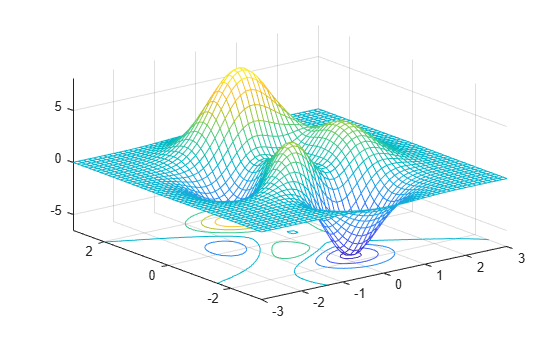Specify the colors for a mesh and contour plot by including a fourth matrix input, C. The plots use Z for height and C for color. Specify the colors using a colormap, which uses single numbers to stand for colors on a spectrum. When you use a colormap, C is the same size as Z. Add a color bar to the graph to show how the data values in C correspond to the colors in the colormap.

[X,Y] = meshgrid(-3:.125:3);
Z = peaks(X,Y);
C = X.*Y;
meshc(X,Y,Z,C)
colorbar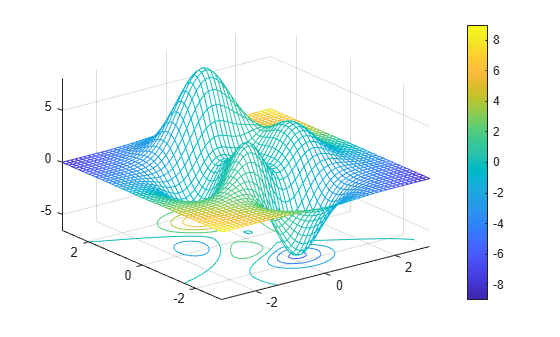Create a mesh plot with a contour plot underneath it. To allow further modifications, assign the graphics array containing the surface and contour objects to the variable sc.

[X,Y] = meshgrid(-5:.5:5);
Z = Y.*sin(X) - X.*cos(Y);
sc = meshc(X,Y,Z);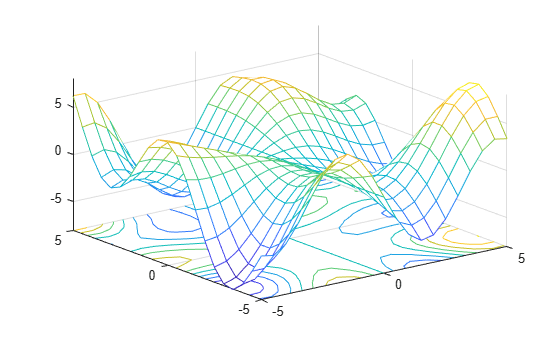Index into sc to access and modify properties of the mesh and contour plots after they are created. The mesh plot is accessible as sc(1) and the contour plot as sc(2). For example, change the edge colors of the two plots by setting the EdgeColor properties.

sc(1).EdgeColor = 'r';
sc(2).EdgeColor = 'b';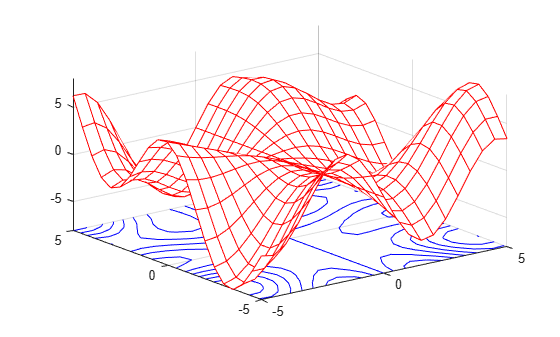The contour lines appear at the minimum z-level by default, but you can change the location by setting the ZLocation property.

Display the peaks data set as a mesh plot with the contours at the minimum z-level. Specify a return argument when you call the meshc function so that you can access the Contour object.

Z = peaks;
sc = meshc(Z);Get the current axes and extend the upper limit of the z-axis to 15. Then move the contours to the maximum z-level.

ax = gca;
ax.ZLim(2) = 15;
sc(2).ZLocation = 'zmax';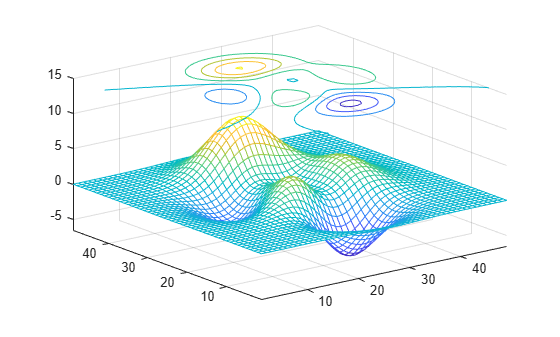Input Arguments

collapse all

x-coordinates, specified as a matrix the same size as Z, or as a vector with length n, where [m,n] = size(Z). If you do not specify values for X and Y, meshc uses the vectors (1:n) and (1:m).

When X is a matrix, the values must be strictly increasing or decreasing along one dimension and remain constant along the other dimension. The dimension that varies must be the opposite of the dimension that varies in Y. You can use the meshgrid function to create X and Y matrices.

When X is a vector, the values must be strictly increasing or decreasing.

The XData properties of the surface and contour objects store the x-coordinates.

Example: X = 1:10

Example: X = [1 2 3; 1 2 3; 1 2 3]

Example: [X,Y] = meshgrid(-5:0.5:5)

Data Types: single | double | int8 | int16 | int32 | int64 | uint8 | uint16 | uint32 | uint64 | categorical

y-coordinates, specified as a matrix the same size as Z or as a vector with length m, where [m,n] = size(Z). If you do not specify values for X and Y, meshc uses the vectors (1:n) and (1:m).

When Y is a matrix, the values must be strictly increasing or decreasing along one dimension and remain constant along the other dimension. The dimension that varies must be the opposite of the dimension that varies in X. You can use the meshgrid function to create X and Y matrices.

When Y is a vector, the values must be strictly increasing or decreasing.

The YData properties of the surface and contour objects store the y-coordinates.

Example: Y = 1:10

Example: Y = [1 1 1; 2 2 2; 3 3 3]

Example: [X,Y] = meshgrid(-5:0.5:5)

Data Types: single | double | int8 | int16 | int32 | int64 | uint8 | uint16 | uint32 | uint64 | categorical

z-coordinates, specified as a matrix. Z must have at least two rows and two columns.

Z specifies the height of the mesh plot at each x-y-coordinate. If you do not specify the colors, then Z also specifies the mesh edge colors.

The ZData properties of the surface and contour objects store the z-coordinates.

Example: Z = [1 2 3; 4 5 6]

Example: Z = sin(x) + cos(y)

Data Types: single | double | int8 | int16 | int32 | int64 | uint8 | uint16 | uint32 | uint64 | categorical

Color array, specified as an m-by-n matrix of colormap indices or as an m-by-n-by-3 array of RGB triplets, where Z is m-by-n.

• To use colormap colors, specify C as a matrix. For each grid point on the mesh surface, C indicates a color in the colormap. The CDataMapping property of the surface object controls how the values in C correspond to colors in the colormap.

• To use truecolor colors, specify C as an array of RGB triplets.

The CData property of the surface object stores the color array. For additional control over the surface coloring, use the FaceColor and EdgeColor properties.

Axes to plot in, specified as an axes object. If you do not specify the axes, then meshc plots into the current axes.

Name-Value Arguments

Specify optional comma-separated pairs of Name,Value arguments. Name is the argument name and Value is the corresponding value. Name must appear inside quotes. You can specify several name and value pair arguments in any order as Name1,Value1,...,NameN,ValueN.

Example: meshc(X,Y,Z,'EdgeColor','red') creates the mesh with red lines.

Note

The properties listed here are only a subset. For a full list, see Surface Properties.

Edges to display, specified as 'both', 'row', or 'column'.

Edge line color, specified as one of the values listed here. The default color of [0 0 0] corresponds to black edges.

ValueDescription
'none'Do not draw the edges.
'flat'

Use a different color for each edge based on the values in the CData property. First you must specify the CData property as a matrix the same size as ZData. The color value at the first vertex of each face (in the positive x and y directions) determines the color for the adjacent edges. You cannot use this value when the EdgeAlpha property is set to 'interp'.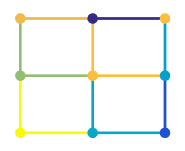'interp'

Use interpolated coloring for each edge based on the values in the CData property. First you must specify the CData property as a matrix the same size as ZData. The color varies across each edge by linearly interpolating the color values at the vertices. You cannot use this value when the EdgeAlpha property is set to 'flat'.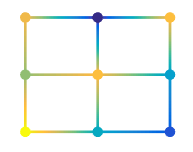RGB triplet, hexadecimal color code, or color name

Use the specified color for all the edges. This option does not use the color values in the CData property.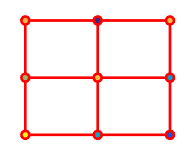RGB triplets and hexadecimal color codes are useful for specifying custom colors.

• An RGB triplet is a three-element row vector whose elements specify the intensities of the red, green, and blue components of the color. The intensities must be in the range [0,1]; for example, [0.4 0.6 0.7].

• A hexadecimal color code is a character vector or a string scalar that starts with a hash symbol (#) followed by three or six hexadecimal digits, which can range from 0 to F. The values are not case sensitive. Thus, the color codes '#FF8800', '#ff8800', '#F80', and '#f80' are equivalent.

Alternatively, you can specify some common colors by name. This table lists the named color options, the equivalent RGB triplets, and hexadecimal color codes.

Color NameShort NameRGB TripletHexadecimal Color CodeAppearance
'red''r'[1 0 0]'#FF0000''green''g'[0 1 0]'#00FF00''blue''b'[0 0 1]'#0000FF''cyan' 'c'[0 1 1]'#00FFFF''magenta''m'[1 0 1]'#FF00FF''yellow''y'[1 1 0]'#FFFF00''black''k'[0 0 0]'#000000''white''w'[1 1 1]'#FFFFFF'Here are the RGB triplets and hexadecimal color codes for the default colors MATLAB® uses in many types of plots.

[0 0.4470 0.7410]'#0072BD'[0.8500 0.3250 0.0980]'#D95319'[0.9290 0.6940 0.1250]'#EDB120'[0.4940 0.1840 0.5560]'#7E2F8E'[0.4660 0.6740 0.1880]'#77AC30'[0.3010 0.7450 0.9330]'#4DBEEE'[0.6350 0.0780 0.1840]'#A2142F'Face color, specified as one of the values in this table.

ValueDescription
'flat'

Use a different color for each face based on the values in the CData property. First you must specify the CData property as a matrix the same size as ZData. The color value at the first vertex of each face (in the positive x and y directions) determines the color for the entire face. You cannot use this value when the FaceAlpha property is set to 'interp'.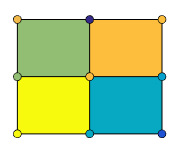'interp'

Use interpolated coloring for each face based on the values in the CData property. First you must specify the CData property as a matrix the same size as ZData. The color varies across each face by interpolating the color values at the vertices. You cannot use this value when the FaceAlpha property is set to 'flat'.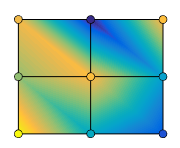RGB triplet, hexadecimal color code, or color name

Use the specified color for all the faces. This option does not use the color values in the CData property.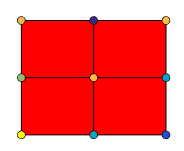'texturemap'Transform the color data in CData so that it conforms to the surface.
'none'Do not draw the faces.

RGB triplets and hexadecimal color codes are useful for specifying custom colors.

• An RGB triplet is a three-element row vector whose elements specify the intensities of the red, green, and blue components of the color. The intensities must be in the range [0,1]; for example, [0.4 0.6 0.7].

• A hexadecimal color code is a character vector or a string scalar that starts with a hash symbol (#) followed by three or six hexadecimal digits, which can range from 0 to F. The values are not case sensitive. Thus, the color codes '#FF8800', '#ff8800', '#F80', and '#f80' are equivalent.

Alternatively, you can specify some common colors by name. This table lists the named color options, the equivalent RGB triplets, and hexadecimal color codes.

Color NameShort NameRGB TripletHexadecimal Color CodeAppearance
'red''r'[1 0 0]'#FF0000''green''g'[0 1 0]'#00FF00''blue''b'[0 0 1]'#0000FF''cyan' 'c'[0 1 1]'#00FFFF''magenta''m'[1 0 1]'#FF00FF''yellow''y'[1 1 0]'#FFFF00''black''k'[0 0 0]'#000000''white''w'[1 1 1]'#FFFFFF'Here are the RGB triplets and hexadecimal color codes for the default colors MATLAB uses in many types of plots.

[0 0.4470 0.7410]'#0072BD'[0.8500 0.3250 0.0980]'#D95319'[0.9290 0.6940 0.1250]'#EDB120'[0.4940 0.1840 0.5560]'#7E2F8E'[0.4660 0.6740 0.1880]'#77AC30'[0.3010 0.7450 0.9330]'#4DBEEE'[0.6350 0.0780 0.1840]'#A2142F'Edge transparency, specified as one of these values:

• Scalar in range [0,1] — Use uniform transparency across all of the edges. A value of 1 is fully opaque and 0 is completely transparent. Values between 0 and 1 are semitransparent. This option does not use the transparency values in the AlphaData property.

• 'flat' — Use a different transparency for each edge based on the values in the AlphaData property. First you must specify the AlphaData property as a matrix the same size as the ZData property. The transparency value at the first vertex determines the transparency for the entire edge. The EdgeColor property also must be set to 'flat'.

• 'interp' — Use interpolated transparency for each edge based on the values in AlphaData property. First you must specify the AlphaData property as a matrix the same size as the ZData property. The transparency varies across each edge by interpolating the values at the vertices. The EdgeColor property also must be set to 'interp'.

Line style, specified as one of the options listed in this table.

Line StyleDescriptionResulting Line
'-'Solid line'--'Dashed line':'Dotted line'-.'Dash-dotted line'none'No lineNo line

Line width, specified as a positive value in points, where 1 point = 1/72 of an inch. If the line has markers, then the line width also affects the marker edges.

The line width cannot be thinner than the width of a pixel. If you set the line width to a value that is less than the width of a pixel on your system, the line displays as one pixel wide.

Tips

• To remove hidden lines from the plot, use the hidden function.

• To control the color shading of the plot surfaces, use the shading function.